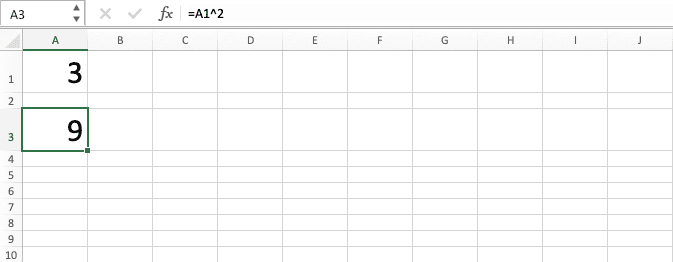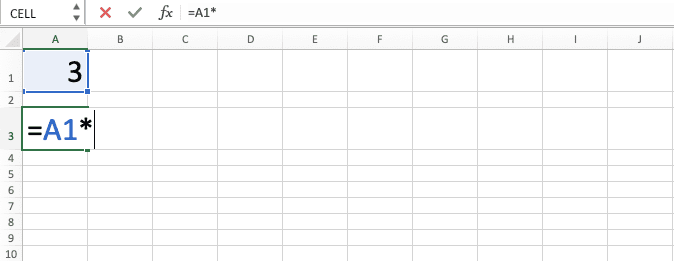How to Make a Square Excel Calculation and All Its Formulas & Functions - Compute Expert

# How to Make a Square Excel Calculation and All Its Formulas & Functions

Home >> Excel Tutorials from Compute Expert >> Excel Calculations >> How to Make a Square Excel Calculation and All Its Formulas & Functions

In this tutorial, we will discuss completely how to make a square excel calculation on a number. All formulas/functions we can use to calculate square in excel will also be explained here.

One calculation we often do when processing numbers is square/the power of two. If we often use excel as a tool to process our numbers, then understanding the way to square there becomes important.

Generally, the method to write a square formula in excel can be divided into three. They are the manual writing with the ^ symbol, manual writing with the * symbol, and the POWER formula. We will talk in the next parts about the general writing form for each, example, and their writing steps in detail.

Disclaimer: This post may contain affiliate links from which we earn commission from qualifying purchases/actions at no additional cost for you. Learn more

## How to Write a Square Excel Calculation With the ^ Symbol

Now, we will discuss the first method to do a square excel calculation, which is by using the ^ symbol.

The caret symbol (^) is a power calculation symbol in excel formula writing. Because the square is the same as the power of 2, we can use it to get a square calculation result.

Generally, here is the writing to calculate a square in excel with the ^ symbol.

=number ^ 2

The number there is the number you want to square/power by 2 in excel. The number input in the formula can be typed or inputted through the media of a cell coordinate.

We use ^ in the writing as a power symbol and 2 as the power factor. We use 2 there because what we look for is the square or the power of 2 from our number.

Write that writing form correctly in your cell and enter. You will immediately get the square result of your number!

To make it clearer about the writing, see its implementation example in excel below.You can see the writing example in the screenshot’s formula bar. We get the square of all the numbers on the left using that form of writing.

To make you understand further about this method to write a square formula, we will give the detailed writing steps here. We will explain each step with the help of a screenshot to make you understand it easier.

1. Type an equal sign ( = ) in the cell where you want to put the square result of your number2. Input the number to be squared or the cell coordinate where it is after =. Type a caret symbol ( ^ ) after that3. Type 24. Press Enter
5. The process is done!## How to Write a Square Excel Calculation With the * Symbol

There is another method for us to write a square calculation formula in excel. That method is using the help of the * symbol.

As we know, the power calculation is the same as multiplying a number with the number itself. Because of that, we can multiply our number with its own value to get its square result in excel.

The symbol that represents multiplication in excel is the star symbol or *. Therefore, we can square our number by using the * symbol in the writing that we do.

Generally, here is the writing form of a square formula in excel with the * symbol.

=number * number

The number there is the number we want to square in our data processing.

To make it clearer about this square formula writing with the * symbol, here is its implementation example in excel.As we can see in the screenshot’s formula bar, the writing in the example uses the form explained earlier. Write the multiplication formula correctly and you can get the square result of your number easily!

1. Type an equal sign ( = ) in the cell where you want to put the square result of your number2. Input the number to be squared or the cell coordinate where it is after =. Type a star symbol ( * ) after that3. Input the number or the cell coordinate again4. Press Enter
5. The process is done!## How to Make a Square Excel Calculation With the POWER Formula

What if we want to use a built-in formula available in excel to square our number? This can be done by using the POWER formula.

POWER is made by excel to calculate the power of a number in our worksheet. The inputs it needs are the number we want to power and the power factor. By inputting 2 in its power factor input, we can get the square result of our number using this formula.

Generally, here is the writing form of POWER so we can get our square calculation result from it.

=POWER(number, 2)

Don’t forget to input 2 in the power factor input part so you can get the correct result.

The implementation example of the writing in excel is as follows.As you can see, POWER can be used to do your number’s square calculation. Give your inputs correctly and you can get your result fast!

To add to your understanding of this method, here are the writing steps.

1. Type an equal sign ( = ) in the cell where you want to put the result in2. Type POWER (can be with large and small letters) and an open bracket sign after =3. Input the number to be squared or the cell coordinate where it is after the open bracket sign. Then type a comma sign ( , )4. Type 25. Type a close bracket sign6. Press Enter
7. The process is done!## Exercise

After learning the methods to write a square formula in excel, now let’s do an exercise. This is so you can deepen your understanding of those methods.

### Questions

1. What is the final score of each person? Use the ^ symbol method to find the answer!
2. What is the final score of each person? Use the * symbol method to find the answer!
3. What is the final score of each person? Use the POWER formula method to find the answer!

To do the power calculation with other factors besides 2, you can learn it by visiting this Compute Expert tutorial.

Related tutorials you should learn:

Get updated excel info from Compute Expert by registering your email. It's free!